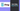# How to Convert integer to string type in Go?

16 Feb, 2023, 3 min read

# How to Convert integer to string type in Go?

You can convert integer to string type in Go using the `Itoa()` function from the `strconv` package. This is helpful when you want to format your integer value into a sentence to log or print the value.

There are two main methods to use to convert integers to string

1. `Itoa()` function from `strconv` package can be used for most cases when dealing with integers of base 10 (Decimal number system) - Recommended for most scenario
2. `FormatInt()` function from `strconv` package can be used when the base of the integer is not 10 and you can specify the base of the integer to calculate the string value.

## Convert integer to string using Itoa() - Method 1

``````package main

import (
"fmt"
"strconv"
)

func main() {
totalMarks := 320
totalMarksStr := strconv.Itoa(totalMarks)
fmt.Printf("The student has scored a total mark of %s out of 500", totalMarksStr)
}``````

### Output

``The student has scored a total mark of 320 out of 500``

## Syntax of the Itoa() function

``func Itoa(i int) string``

Itoa() function represents the conversion of integer to string and is part of the `strconv` package. The function takes an integer as an argument and returns a string value

Itoa() function returns the equivalent to FormatInt(int64(i), 10). You can learn more about the FormatInt() function in the next section which is the advanced way of converting integers that are not base 10.

## Convert integer to string using FormatInt - Method 2

`FormatInt` function is a more advanced way to format your Integer to string value. You can send the integer as the first argument and the second argument will be the base for the integer. If you want to convert the integer into a normal decimal numerical system of numbers from 0 to 9, you can send the base value as `10`.

If you want to get the string of binary representation of the integer, you can pass the value of the base as `2`

``````package main

import (
"fmt"
"strconv"
)

func main() {
totalMarks := 320
totalMarksStr := strconv.FormatInt(int64(totalMarks), 10)
fmt.Printf("The student has scored a total mark of %s out of 500", totalMarksStr)
}``````

`int64` function is used to convert the int type into an integer with 64 bits. In this case, since the `FormatInt` first argument is expecting the integer in `int64`, you will be converting the normal integer which is a 32-bit to a 64-bit integer.

### Output

``The student has scored a total mark of 320 out of 500``

## Syntax of ParseInt() function

``func FormatInt(i int64, base int) string``

The first argument is the integer which will be used to string based on the second argument. The second argument is the base which is the numeric base to convert. When converting to a decimal system (digits from 0 to 9), you can use the base of `10`. Base 10 will be most used since most of the applications will be working on a Decimal numerical system

## Conversion of integer to binary string in Golang

If you want to represent the integer in a binary string, you can use the base of `2`

``````package main

import (
"fmt"
"strconv"
)

func main() {
totalMarks := 320
totalMarksStr := strconv.FormatInt(int64(totalMarks), 10)
fmt.Printf("The student has scored a total mark of %s out of 500\n", totalMarksStr)

totalMarkBinary := strconv.FormatInt(int64(totalMarks), 2)
fmt.Println("Binary - ", totalMarkBinary)
}``````

### Output

``````The student has scored a total mark of 320 out of 500
Binary -  101000000``````

## Conversion of integer to hexadecimal string in Golang

When you want to represent the integer in hexadecimal format, you can use the `FormatInt` function to get the hexadecimal string. This can be useful when you want to know the hex value of a specific channel in the RGB value of the colors.

``````package main

import (
"fmt"
"strconv"
)

func main() {
redColorValue := 142
hexValue := strconv.FormatInt(int64(redColorValue), 16)
fmt.Println("Hex Value -", hexValue)
}``````

When the color of the red channel is 142, then the corresponding Hex value of the color will start with `8e`, so it is easy to understand how the integer value is converted to hexadecimal.

### Output

``Hex Value - 8e``

Golang Maps

Golang Switch